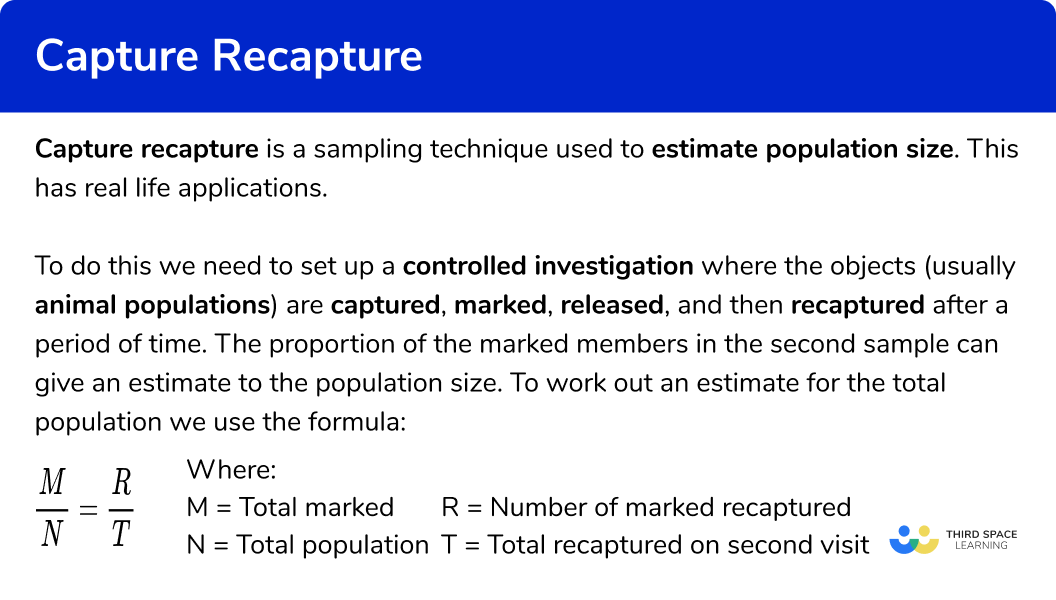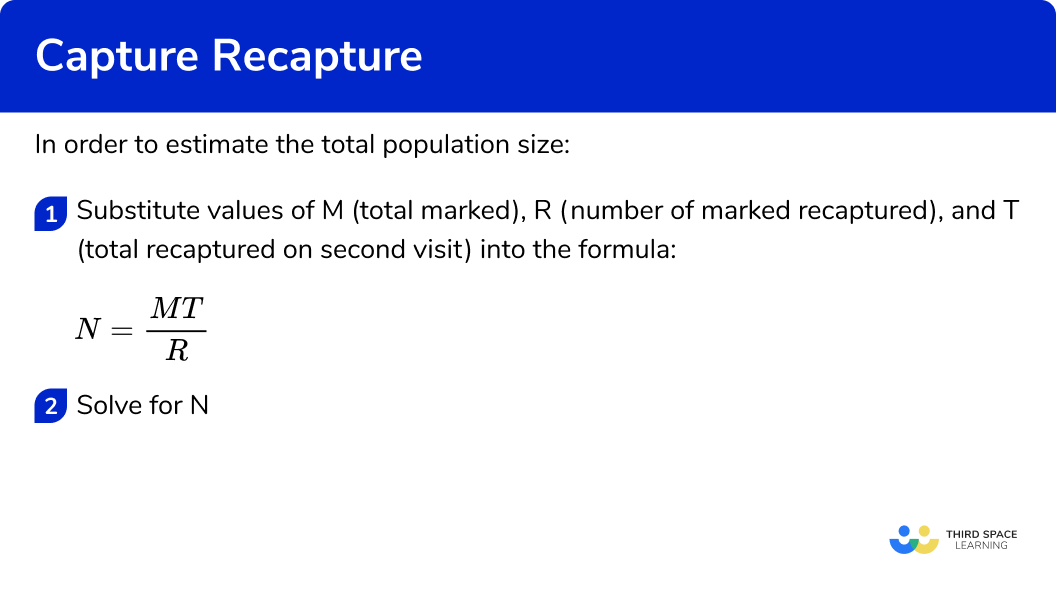# Capture Recapture

Here we will learn about capture recapture, including what capture recapture is, how to use capture recapture and the advantages and disadvantages of this method.

There are also types of sampling worksheets based on Edexcel, AQA and OCR exam questions, along with further guidance on where to go next if you’re still stuck.

## What is capture recapture?

Capture recapture is a sampling technique used to estimate population size.

To do this we need to set up a controlled investigation where the objects (usually animal populations) are captured, marked, released, and then recaptured after a period of time. The proportion of the marked members in the second sample can give an estimate to the population size.

Sampling methodDescriptionExample
Capture recaptureCollecting a sample data from one location at different points in time, marking individuals to estimate a population sizeA sample of woodlice were captured, marked and released. Another sample of woodlice was captured 5 days later and the number of marked woodlice was counted.

Estimate population size Track population changes (e.g. seasonal, health, climate change) Tracking over time.Individuals have to remain local to the area of research with a definite boundary (no radical changes in the population due to births / deaths / migration). Markers are not lost or removed.

Capture recapture studies and capture recapture analysis can help to determine the spread of disease, conservation efforts, the number of animals in a population, and many more real life applications in ecology, epidemiology, and population biometrics.

## Calculating the total population using capture recapture

To work out an estimate for the total population we use the formula:

\frac{M}{N}=\frac{R}{T}

where:

M = Total marked

N = Total population

R = Number of marked recaptured

T = Total recaptured on second visit

This can be rearranged using basic algebraic principles to obtain the formula:

N=\frac{MT}{R}

E.g.
A farmer wants to calculate the number of mice in a barn.

One day he places a trap for mice to enter. 12 mice are counted, marked, and released. 5 days later, he places the same trap down for mice to enter. This time he counts 14 mice, 7 of which have been marked from the previous capture. The mice were then released.

Estimate the total size of the mouse population.

Here we are estimating the value of N (the total population):

M = 12 (the total marked on the first capture)

R = 7 (the total marked on the second capture)

T = 14 (the total captured on the second capture)

So we now have:

N=\frac{12\times{14}}{7}=24

The estimate for the population of mice in the barn is 24 .

This is because 7 out of the 14 mice in the recapture were already marked, which is 50% of the sample and so if 12 mice represented 50% of the population, 100% would be twice this amount, which is 24 .

## Assumptions of capture recapture

There are several assumptions that have to be made when using the capture recapture methodology and study design:

• There must be enough time and opportunity for the original captured sample to mix with the population
• The marking doesn’t effect the individual’s chance of survival, but is still visible
• It must be a closed population. This means there is no change in population size during the period of the study (births and deaths, migration etc)

### What is capture recapture?## How to find the total population size using capture recapture

In order to estimate the total population size:

1. Substitute values of M (total marked), R (number of marked recaptured), and T (total recaptured on second visit) into the formula N=\frac{MT}{R}
2. Solve for N .

### How to find the total population size using capture recaptureCapture recapture is part of our series of lessons to support revision on types of sampling methods. You may find it helpful to start with the main types of sampling methods lesson for a summary of what to expect, or use the step by step guides below for further detail on individual topics. Other lessons in this series include:

## Capture recapture examples

### Example 1: capture recapture

Alan is researching the population of woodlice in his garden. He sets up a trap and collects 36 woodlice over one night. He marks them using UV paint, and then releases them back into his garden. One week later, he repeats the experiment by placing the same trap in the same location to collect the woodlice over the same time frame. The second sample he collected had 20 woodlice. Of these, 8 had the original UV paint marking. Estimate the population size of woodlice in Alan’s garden.

1. Substitute values of M (total marked), R (number of marked recaptured), and T (total recaptured on second visit) into the formula N=\frac{MT}{R}

We know that, M=36, R=8, and T=20 . Substituting these values into the formula, we get:

N=\frac{MT}{R}=\frac{36\times{20}}{8}

2Solve for N

N=\frac{720}{8}=90

The estimated population size of woodlice in Alan’s garden is 90 .

### Example 2: capture recapture

Rebecca is researching the number of rabbits in a field. The rabbits were captured over one week and placed in a secure habitat before being marked and released back to where they were found. One month later, she repeated the experiment. Below is a table showing the results of her experiment including the recapture data.

Determine an estimate for the overall population size.

Substitute values of M (total marked), R (number of marked recaptured), and T (total recaptured on second visit) into the formula N=\frac{MT}{R}

Solve for N

### Example 3: capture recapture

Charlie owns a fishing lake. He wants to find out approximately how many fish are in the lake. He decides to set up a trap which captures the fish and places them in a separate enclosure for 24 hours. He marks each of the fish he captured and then releases them back into the lake. 5 days later, he repeats the same experiment over the same time interval. He then counts how many fish have the original marking from the previous capture, and releases them back into the lake. Below is a table of the findings.

Calculate an estimate for the population size of fish in the lake.

Substitute values of M (total marked), R (number of marked recaptured), and T (total recaptured on second visit) into the formula N=\frac{MT}{R}

Solve for N

### Example 4: capture recapture

A wildlife study is being carried out on the population of flamingos in the nature reserve. Flamingos are captured, placed in a separate habitat for 3 hours, marked using a small ring around their leg, and then released back into the population. The size of their initial sample captured was 240 flamingos. The next day, they repeat the experiment. They recaptured 200 flamingos during the same time period and counted 40 with rings. The flamingos were then released back into the reserve. Find the estimate for the total number of flamingos in the total population (flamboyance).

Substitute values of M (total marked), R (number of marked recaptured), and T (total recaptured on second visit) into the formula N=\frac{MT}{R}

Solve for N

### Common misconceptions

• Incorrect value for R (the number of marked items recaptured)

The number of marked items in sample 2 cannot exceed the number captured in either sample, so R has to be the smallest of the three values.

• Rearranging the formula incorrectly

The formula for an estimate of the population uses proportionality. It estimates the population size given the proportion of recaptured items from the second sample. If you use the formula \frac{M}{N}=\frac{R}{T} you could simplify the fraction of \frac{R}{T} first and use equivalent fractions to find the value of N .

• Population size and sample size

Context for these questions is very important. A sample size can be less than or equal to the population size, never greater than by definition.

### Practice capture recapture questions

1. Using the information in the table below, determine an estimate for the population size of bats85,58037,1802,98640,456N=\frac{MT}{R}=\frac{1430}{1556}{26}=85,580

2. 52 Bison were captured, marked using a tracking collar and released back into the herd over 2 hours. After one day, a second sample of 73 bison were captured, of which 60 did not have a tracking collar. Estimate the population size of bison in the herd.

125292676949N=\frac{MT}{R}=\frac{52\times{73}}{13}=292

3. A biologist is studying the number of springboks that attended the annual rut. On one day, he captured, marked and released 20 springboks. The next day, he captured 32 , of which 6 had the original marking made the day before. How many springboks are estimated to have attended the rut?

107106192120N=\frac{MT}{R}=\frac{20\times{32}}{6}=107

4. A kaleidoscope of butterflies was being investigated by a lepidopterist. She managed to capture 431 butterflies over a 2 hour period. Each butterfly was marked with UV paint and released back into nature. 2 days later, she repeats the experiment. She counts only 3 butterflies with the original UV marking out of the 387 she captured. Estimate the population size of the kaleidoscope.

56,030166,79755,16855,599N=\frac{MT}{R}=\frac{431\times{387}}{3}=55,599

### Capture recapture GCSE questions

1. Roland is researching the population of hedgehogs in the local woodland. Over one week, he collects 13 hedgehogs, marks them using a ring and then releases them back into the same woodland. One year later, he returns to repeat the experiment. He captures 20 hedgehogs, of which 12 had the original rings from the previous capture.

(a) Estimate the population size of the hedgehogs in the woodland. Show your working.

(b) Explain why Roland’s data may not be very reliable.

(5 marks)

(a)

N=\frac{MT}{R}

(1)

N=\frac{13\times{20}}{12}=21.66…

(1)

22

(1)

(b)

Too much time passed between both samples

(1)

Births / deaths / migration may affect population size

(1)

2. (a) John estimates that the population of snails in his garden is around 100 . If he captured, marked and released 20 snails in one night, and captured 28 snails one week later, how many of the snails in the second sample should have a mark?

(b) John finds that 18 snails were marked in his second sample. How does this affect his estimate on the total number of snails in his garden? Explain your answer.

(5 marks)

(a)

M=20, N=100, T=28

(1)

R=\frac{MT}{N}=\frac{20\times{28}}{100}=5.6

(1)

6 should be marked in sample 2

(1)

(b)

N=\frac{MT}{R}=\frac{20\times{28}}{18}=31  to the nearest integer

(1)

As more snails were marked in sample 2 , the proportion of marked to unmarked snails in sample 2 is smaller, reducing the overall population total.

(1)

3. Laura tracks the population of limpets on a beach over 3 days. She marks each limpet in sample 1 and records her findings in a table:Using the data in the table, estimate the total population of limpets in Laura’s study.

(3 marks)

N=\frac{MT}{R}

(1)

\frac{38\times{47}}{32}=55.8125

(1)

56 limpets

(1)

## Learning checklist

You have now learned how to:

• Infer properties of populations or distributions from a sample, whilst knowing the limitations of sampling

## Still stuck?

Prepare your KS4 students for maths GCSEs success with Third Space Learning. Weekly online one to one GCSE maths revision lessons delivered by expert maths tutors.

Find out more about our GCSE maths tuition programme.